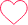# Find the Sum of Numbers in a given Range in Python## Find the Sum of the Numbers in a Given Range

Given two integer inputs as the range [ low , high ], the objective is to find the sum of the numbers that lay in the intervals given by the integer inputs. Therefore we’ll write a code to Find the Sum of the Numbers in a Given Range in Python Language.

```Example
Input : 2 5
Output : 14
```

## Find the Sum of the Numbers in a Given Interval in Python Language

Given two integer inputs as the range [low , high], the objective is to find the sum of all the numbers that lay in the given integer inputs as interval. In order to do so we usually iterate through the the numbers in the given range and keep appending them to the sum variable. Here are few methods to solve the above mentioned problem in Python Language.

• Method 1: Using Brute Force
• Method 2: Using the Formula
• Method 3: Using Recursion

We’ll discuss the above mentioned methods in detail in the sections below.

### Method 1: Using Brute Force

In this method we’ll use loops like for, while and do while to sum all the numbers that lay in the intervals of the given input integers.

### Working

For in integer inputs num1 and num2 as the intervals

• Initialize the required variables.
• Run a for loop from num1 to num2+1 i.e [num1,num2].
• Append i to sum variable with each iteration.
• Print sum variable.

Let’s implement the above logic in Python Language.

### Python Code

```num1, num2 = 3, 6
sum = 0
for i in range(num1,num2+1):
sum+=i
print(sum)
```

```18
```

### Method 2: Using the Formula

In this method we’ll use formula mentioned below to find the sum of all the numbers that lay in the interval given by the input variable.

### Working

For the two integer inputs num1 and num2

• Initialize the required variables.
• perform sum = int((num2*(num2+1)/2) – (num1*(num1+1)/2) + num1).
• Print Sum variable.

Let’s implement the above logic in Python Language.

### Python Code

```num1, num2 = 3, 6
sum = int((num2*(num2+1)/2) - (num1*(num1+1)/2) + num1)
print(sum)
```

```18
```

### Method 3: Using Recursion

In this method we’ll use recursion to find the sum of all the numbers that lay in the interval given by the input variable. To know more about recursion, refer to Recursion in Python.

### Working

For the two integer inputs num1 and num2 as the interval

• Initialize the required variables.
• Define a recursive function with base case as num1>num2.
• Set the recursive step call as num1 + recursum(sum, num1+1, num2).
• call the recursum() function and print the returned value.

Let’s implement the above logic in Python Language.

### Python Code

```def recursum(sum,num1,num2):
if num1 > num2:
return sum
return num1 + recursum(sum,num1+1,num2)

num1, num2 = 3, 6
sum = 0
print(recursum(sum,num1,num2))
```

```18
```

### Related Banners

Get PrepInsta Prime & get Access to all 200+ courses offered by PrepInsta in One Subscription

## Get over 200+ course One Subscription

Courses like AI/ML, Cloud Computing, Ethical Hacking, C, C++, Java, Python, DSA (All Languages), Competitive Coding (All Languages), TCS, Infosys, Wipro, Amazon, DBMS, SQL and others

## Getting Started

### One comment on “Find the Sum of Numbers in a given Range in Python”

•Raghvendra Singh Shekhawat

N1 = int(input(“Enter the first number : “))
N2 = int(input(“Enter the second number : “))

sum = 0

for i in range(N1,N2+1):
sum += i
print(f”sum is {sum}”)0